# Variable Transformations

There are two main variable transformations in the code:

2. Diminishing returns

This technique is very useful for the better and more accurate representation of the real carryover effect of marketing campaigns. Moreover, it helps to improve the understanding of decay effects and how this can be used in campaign planning. It reflects the theory that the effects of advertising can lag and decay following initial exposure. In other words, not all effects of advertising are felt immediately—memory builds and people sometimes delay action—and this awareness diminishes as time passes.

There are two adstock techniques you may choose from the code:

1. Geometric: Traditionally the exponential decay function is used and on data controlled by theta the decay parameter. For example, an ad-stock of theta = 0.75 means that 75% of the impressions in Period 1 were brought to Period 2. Mathematically the traditional exponential adstock decay effect is defined as: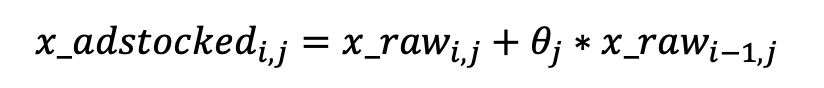Below you will find the adstock geometric function within the code. Please bear in mind that all functions are located within the ‘func.R’ script.

``#### Define adstock geometric functionf.adstockGeometric <- function(x, theta) {  x_decayed <- c(x ,rep(0, length(x)-1))  for (xi in 2:length(x_decayed)) {    x_decayed[xi] <- x[xi] + theta * x_decayed[xi-1]  }  return(x_decayed)}``
1. Weibull: Weibull survival function (Weibull distribution) provides much more flexibility in the shape and scale of the distribution. The formula is defined as: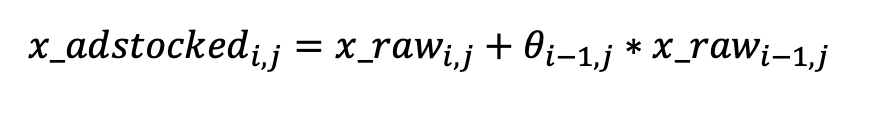This is the function within the code for weibull:

``#### Define adstock weibull functionf.adstockWeibull <- function(x, shape , scale) {  x_bin <- 1:length(x)  x_decayed <- c(x ,rep(0, length(x)-1))  for (xi in 2:length(x_decayed)) {    theta <- 1-pweibull(x_bin[xi-1], shape = shape, scale = scale)    x_decayed[xi] <- x[xi] + theta * x_decayed[xi-1]  }  return(x_decayed)}``

## Diminishing returns#

The theory of diminishing returns holds that each additional unit of advertising increases the response, but at a declining rate. This key marketing principle is reflected in marketing mix models as a variable transformation.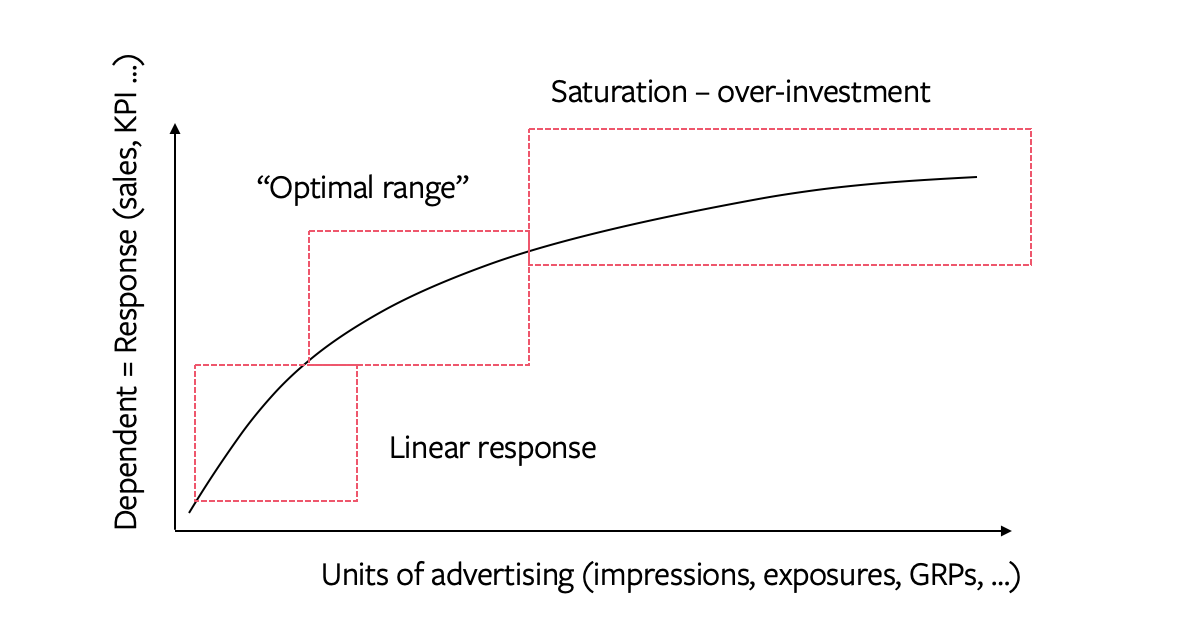The nonlinear response to a media variable on the dependent variable can be modelled using a variety of functions. For example, we can use a simple logarithm transformation (taking the log of the units of advertising log(x) ), or a power transformation (x^alpha). In the case of a power transformation, the modeler tests the different variables (different levels of parameter x) for the highest significance of the variable in the model and the highest significance of the equation overall. However, the most common approach is to use the flexible S-curve transformation: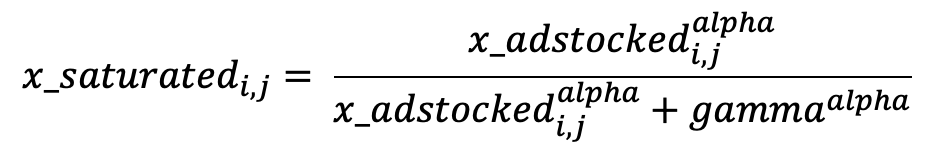The variations of the parameters give modelers full flexibility on the look of the S-curve, specifically the shape and the inflection points: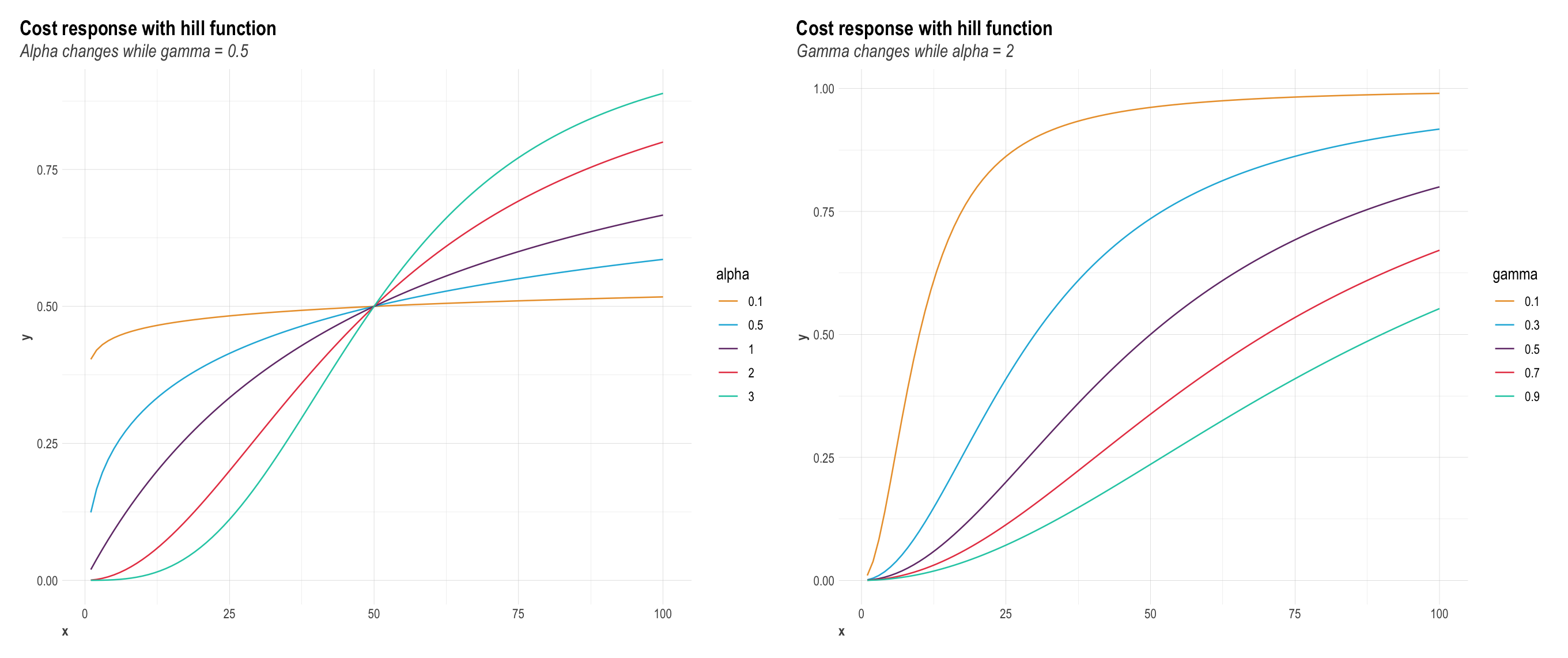This is the function within the code for Diminishing Returns (Hill function):

``## step 3: s-curve transformationgammaTrans <- round(quantile(seq(range(x_normalized), range(x_normalized), length.out = 100), gamma),4)x_scurve <-  x_normalized**alpha / (x_normalized**alpha + gammaTrans**alpha)``